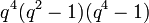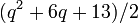# Element structure of projective symplectic group of degree four over a finite field

## Contents

This article gives specific information, namely, element structure, about a family of groups, namely: projective symplectic group of degree four.
View element structure of group families | View other specific information about projective symplectic group of degree four

This article describes the element structure of the projective symplectic group of degree four over a finite field of size$q$, denoted$PSp(4,q)$. This group is a Chevalley group of type C, and with the Chevalley notation, it is denoted$C_2(q)$. Note that the parameter as a Chevalley group is half the order of the matrices.

## Summary

Item Value
order of the group Case$q$ even (e.g.,$q = 2,4,8,16,\dots$):$q^4(q^2 - 1)(q^4 - 1)$
Case$q$ odd (e.g.,$q = 3,5,7,9,11,\dots$):$q^4(q^2 - 1)(q^4 - 1)/2$
number of conjugacy classes Case$q$ a power of 2 (e.g.,$q = 2,4,8,16,\dots$):$q^2 + 2q + 3$
Case$q$ odd (e.g.,$q = 3,5,7,9,11,\dots$):$(q^2 + 6q + 13)/2$

## Number of conjugacy classes

The general theory tells us that number of conjugacy classes in projective symplectic group of fixed degree over a finite field is PORC function of field size. For$PSp(2m,q)$, the degree of the polynomial is$m$ and the polynomial depends on the value$\operatorname{gcd}(\operatorname{lcm}(2,m),q-1)$. In this case,$m = 2$, so the polynomials have degree two and they depend on$\operatorname{gcd}(2,q-1)$. This is exactly what happens:

Value of$\operatorname{gcd}(2,q-1)$ Corresponding congruence classes mod 2 Number of conjugacy classes (polynomial of degree 2) Additional comments
1 0 (e.g.,$q = 2,4,8,\dots$)$q^2 + 2q + 3$ In this case, the projective symplectic group coincides with the symplectic group, because the symplectic group is centerless
2 1 (e.g.,$q = 3,5,7,9,\dots$)$(q^2 + 6q + 13)/2$